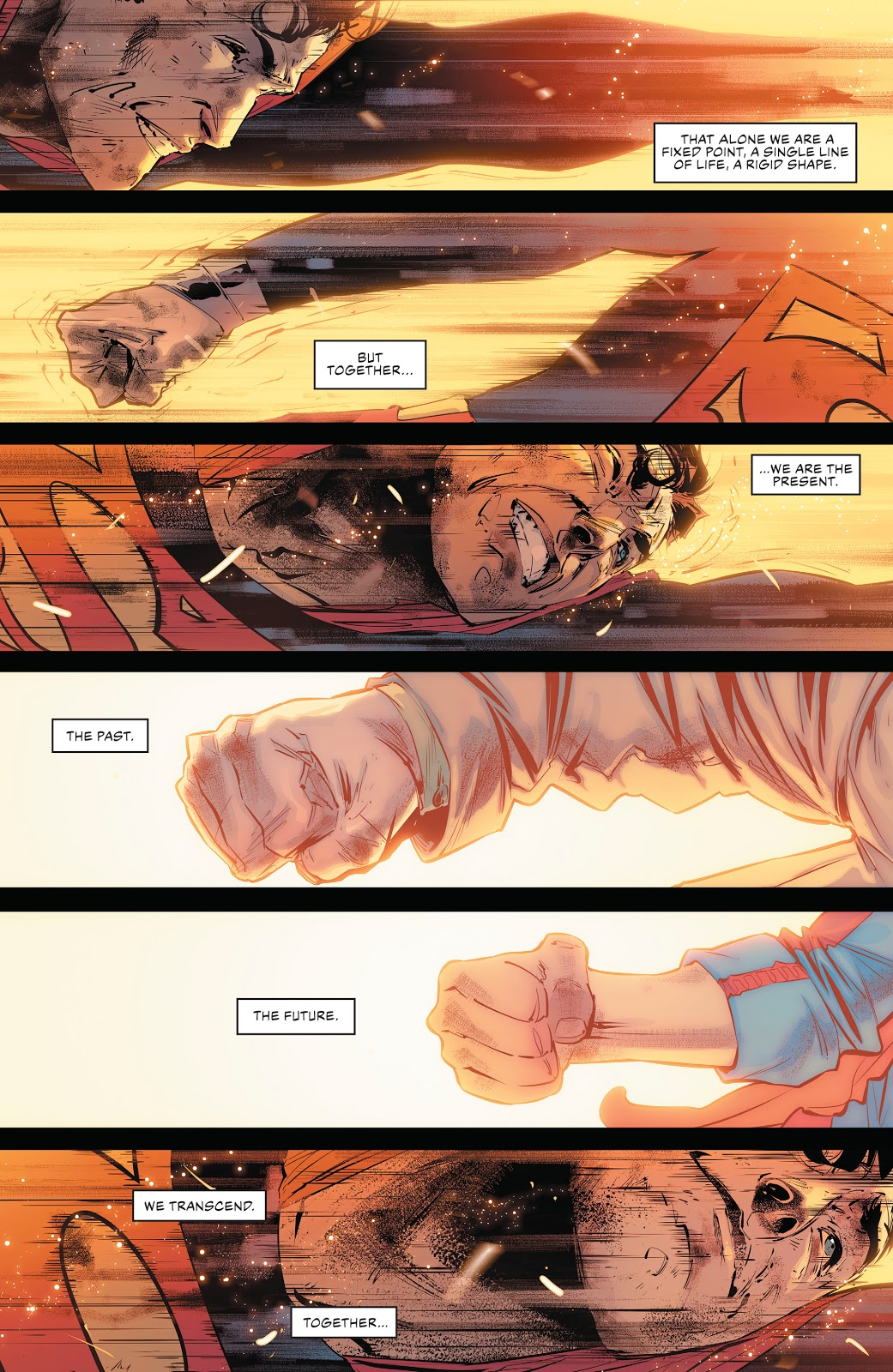Posted on

# Feat: Superman, ‘Justice League’ Vol 4 #25## IN COSMIC HIERARCHY

In Justice League (2019) #25, titled “The Sixth Dimension! Conclusion,” Superman performs a super strength feat. In a battle against the World Forger, Superman sundips then breaches the Sixth Dimension and shatters the Forger’s Multiverse creation with a dimension-shattering punch.In Justice League (2019) #25, titled “The Sixth Dimension! Conclusion,” Superman performs a super strength feat. In a battle against the World Forger, Superman breaches the Sixth Dimension and shatters the Forger’s Multiverse creation with a punch. AP Photo/DC Comics.

## MULTIVERSAL

For more DC feats, visit the DC Power Levels page!

Superman travels faster than light from his prison on Prime Earth to the Sixth Dimension. Superman travels so fast that he becomes a line where all timelines are present to him and he arrives at the time of a new Big Bang. Superman destroys the World Forger’s Sixth Dimension Multiverse creation with a dimension-shattering punch.

We will use string theory to calculate the energy necessary to travel to the Sixth Dimension according to the Many-Worlds Interpretation. Please Toggle the Toggle Arrow below to see the calculations.

#### NUMBER CRUNCHING

In order to calculate the energy necessary to travel to the Sixth Dimension, we must calculate the universal wavefunction interpretation of string theory. According to Justice League (2019) #22, the multiverse created by Perpetua and her three beings is 20 billion years old. We can assume the World Forger in the Sixth Dimension created the Multiverse with a Big Bang 20 billions years old. In order to dimension travel to the Sixth Dimension, Superman had to travel faster than light 20 billion years old into the past of the DC Universe. For all intents and purposes, we can assume Superman travelled at the speed of light. The equation to calculate the wave function is below:

ψ(L,T) = exp(i ʃ0Tdτ ʃ0 Ldσ/lptp) ψ(0,0).

Integrating the double integral we obtain the integral of the inside function first:

ψ(L,T) = exp(i ʃ0Tdτ (σ/lptp)|0 1.8662410^26 = exp(i ʃ0Tdτ (1.8662410^26 m)/(1.616*10^-35 m * 5.39*10^-44 s).

Then we obtain the integral of the outside function.

ψ(L,T) = exp(i ʃ0 9.331210^15 (τ)(2.142582e+104 s^-1) = exp(9.331210^15 s)(2.142582e+104 s^-1) = ∞.

We get an infinite amount of energy.

#### SUPER-STRENGTH

Superman generated an infinite amount of energy in order to become a wave function at the Planck scale and time travel to the Sixth Dimension.

Superman had to generate a Multiversal Level of Super Strength since he essentially hit the World Forger’s Multiverse with an infinite amount of energy from time travel to the Big Bang.

Toggle the Toggle Arrow below to view the calculations.

Calculations for Superman, ‘Justice League’ #25

ψ(L,T) = exp(i ʃ0Tdτ ʃ0 Ldσ/lptp) ψ(0,0).
Planck length = lp = 1.616*10^-35 m.
Planck time = tp = 5.39*10^-44 s.
T = age of causal horizon = (20*10^9 years)(12 months*30 days*24 hr*3600 s) = 9.3312*10^15 s
L = length of causal horizon = vt = (3*10^8 m/s)(9.3312*10^15 s) = 1.86624*10^26 m = 19.7265937*10^9 light years.

ψ(L,T) = exp(i ʃ0Tdτ (σ/lptp)|0 1.86624*10^26 = exp(i ʃ0Tdτ (1.86624*10^26 m)/(1.616*10^-35 m * 5.39*10^-44 s) = exp(i ʃ0Tdτ (2.142582e+104 s^-1) = exp(i ʃ0 9.3312*10^15 (τ)(2.142582e+104 s^-1) = exp(9.3312*10^15 s)(2.142582e+104 s^-1) = ∞.

For the issue, click on the Amazon product image below to purchase the comic.

## 4 thoughts on “Feat: Superman, ‘Justice League’ Vol 4 #25”

1. […] the two contestants at the Grand Canyon. On the left side is Superman, the Man of Steel and on the right side is Hulk, the Green […]

2. […] Journey, Chapter One: The Call for Adventure”, Cyborg performs an intelligence feat. Batman, Superman and Cyborg are trying to stop prison escapes at Arkham Asylum caused by a Batman rogue named the […]

3. […] apprehends the second one and when he jumps down a building to prevent the bomb from detonating, Superman saves him and and catches the other terrorist. Back at the Fortress of Solitude, Dick and Superman […]

4. […] VISIT FEAT: SUPERMAN, ‘JUSTICE LEAGUE’ #25 […]